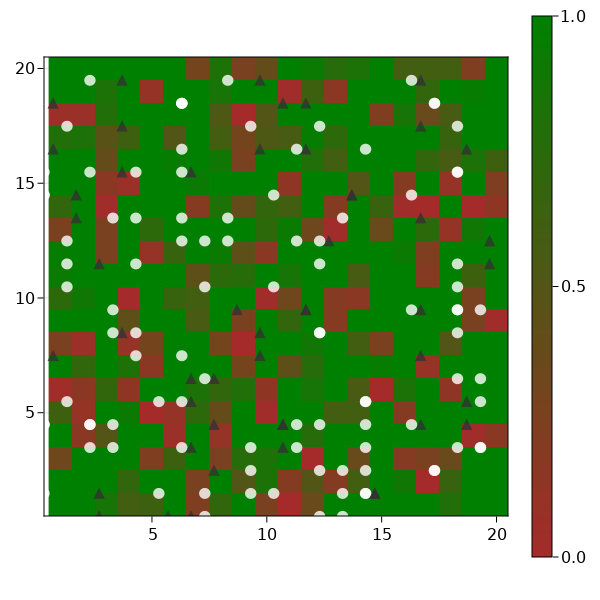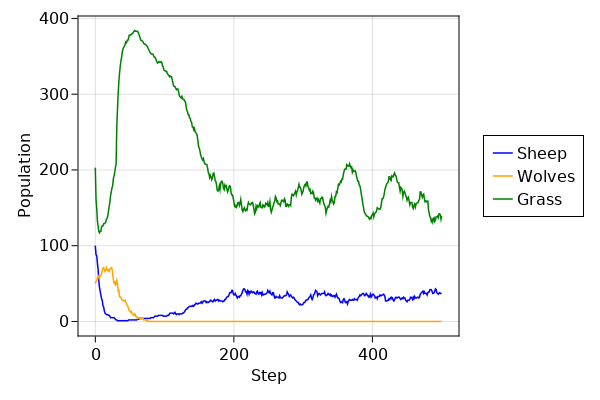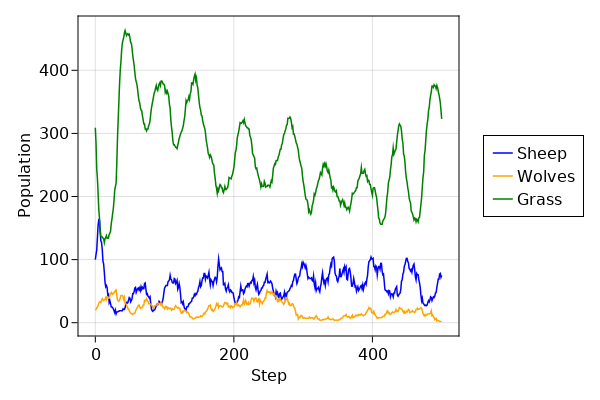# Predator-prey dynamics

The predator-prey model emulates the population dynamics of predator and prey animals who live in a common ecosystem and compete over limited resources. This model is an agent-based analog to the classic Lotka-Volterra differential equation model. This example illustrates how to develop models with heterogeneous agents (sometimes referred to as a mixed agent based model).

The environment is a two dimensional grid containing sheep, wolves and grass. In the model, wolves eat sheep and sheep eat grass. Their populations will oscillate over time if the correct balance of resources is achieved. Without this balance however, a population may become extinct. For example, if wolf population becomes too large, they will deplete the sheep and subsequently die of starvation.

We will begin by loading the required packages and defining three subtypes of AbstractAgent: Sheep, Wolf, and Grass. All three agent types have id and pos properties, which is a requirement for all subtypes of AbstractAgent when they exist upon a GridSpace. Sheep and wolves have identical properties, but different behaviors as explained below. The property energy represents an animals current energy level. If the level drops below zero, the agent will die. Sheep and wolves reproduce asexually in this model, with a probability given by reproduction_prob. The property Δenergy controls how much energy is acquired after consuming a food source.

Grass is a replenishing resource that occupies every position in the grid space. Grass can be consumed only if it is fully_grown. Once the grass has been consumed, it replenishes after a delay specified by the property regrowth_time. The property countdown tracks the delay between being consumed and the regrowth time.

It is also available from the Models module as Models.predator_prey.

using Agents, Random

mutable struct SheepWolf <: AbstractAgent
id::Int
pos::Dims{2}
type::Symbol # :sheep or :wolf
energy::Float64
reproduction_prob::Float64
Δenergy::Float64
end

Simple helper functions

Sheep(id, pos, energy, repr, Δe) = SheepWolf(id, pos, :sheep, energy, repr, Δe)
Wolf(id, pos, energy, repr, Δe) = SheepWolf(id, pos, :wolf, energy, repr, Δe)
Wolf (generic function with 1 method)

The function initialize_model returns a new model containing sheep, wolves, and grass using a set of pre-defined values (which can be overwritten). The environment is a two dimensional grid space, which enables animals to walk in all directions.

function initialize_model(;
n_sheep = 100,
n_wolves = 50,
dims = (20, 20),
regrowth_time = 30,
Δenergy_sheep = 4,
Δenergy_wolf = 20,
sheep_reproduce = 0.04,
wolf_reproduce = 0.05,
seed = 23182,
)

rng = MersenneTwister(seed)
space = GridSpace(dims, periodic = false)
# Model properties contain the grass as two arrays: whether it is fully grown
# and the time to regrow. Also have static parameter regrowth_time.
# Notice how the properties are a NamedTuple to ensure type stability.
properties = (
fully_grown = falses(dims),
countdown = zeros(Int, dims),
regrowth_time = regrowth_time,
)
model = ABM(SheepWolf, space; properties, rng, scheduler = Schedulers.randomly)
id = 0
for _ in 1:n_sheep
id += 1
energy = rand(1:(Δenergy_sheep*2)) - 1
sheep = Sheep(id, (0, 0), energy, sheep_reproduce, Δenergy_sheep)
end
for _ in 1:n_wolves
id += 1
energy = rand(1:(Δenergy_wolf*2)) - 1
wolf = Wolf(id, (0, 0), energy, wolf_reproduce, Δenergy_wolf)
end
for p in positions(model) # random grass initial growth
fully_grown = rand(model.rng, Bool)
countdown = fully_grown ? regrowth_time : rand(model.rng, 1:regrowth_time) - 1
model.countdown[p...] = countdown
model.fully_grown[p...] = fully_grown
end
return model
end
initialize_model (generic function with 1 method)

The function sheepwolf_step! is dispatched on the sheep and wolves similarly: both lose 1 energy unit by moving to an adjacent position and both consume a food source if available. If their energy level is below zero, they die. Otherwise, they live and reproduces with some probability.

Sheep and wolves move to a random adjacent position with the walk! function.

function sheepwolf_step!(agent::SheepWolf, model)
if agent.type == :sheep
sheep_step!(agent, model)
else # then agent.type == :wolf
wolf_step!(agent, model)
end
end

function sheep_step!(sheep, model)
walk!(sheep, rand, model)
sheep.energy -= 1
sheep_eat!(sheep, model)
if sheep.energy < 0
kill_agent!(sheep, model)
return
end
if rand(model.rng) <= sheep.reproduction_prob
reproduce!(sheep, model)
end
end

function wolf_step!(wolf, model)
walk!(wolf, rand, model)
wolf.energy -= 1
agents = collect(agents_in_position(wolf.pos, model))
dinner = filter!(x -> x.type == :sheep, agents)
wolf_eat!(wolf, dinner, model)
if wolf.energy < 0
kill_agent!(wolf, model)
return
end
if rand(model.rng) <= wolf.reproduction_prob
reproduce!(wolf, model)
end
end
wolf_step! (generic function with 1 method)

Sheep and wolves have separate eat! functions. If a sheep eats grass, it will acquire additional energy and the grass will not be available for consumption until regrowth time has elapsed. If a wolf eats a sheep, the sheep dies and the wolf acquires more energy.

function sheep_eat!(sheep, model)
if model.fully_grown[sheep.pos...]
sheep.energy += sheep.Δenergy
model.fully_grown[sheep.pos...] = false
end
end

function wolf_eat!(wolf, sheep, model)
if !isempty(sheep)
dinner = rand(model.rng, sheep)
kill_agent!(dinner, model)
wolf.energy += wolf.Δenergy
end
end
wolf_eat! (generic function with 1 method)

Sheep and wolves share a common reproduction method. Reproduction has a cost of 1/2 the current energy level of the parent. The offspring is an exact copy of the parent, with exception of id.

function reproduce!(agent, model)
agent.energy /= 2
id = nextid(model)
offspring = SheepWolf(
id,
agent.pos,
agent.type,
agent.energy,
agent.reproduction_prob,
agent.Δenergy,
)
return
end
reproduce! (generic function with 1 method)

The behavior of grass functions differently. If it is fully grown, it is consumable. Otherwise, it cannot be consumed until it regrows after a delay specified by regrowth_time. The grass is tuned from a model stepping function

function grass_step!(model)
@inbounds for p in positions(model) # we don't have to enable bound checking
if !(model.fully_grown[p...])
if model.countdown[p...] ≤ 0
model.fully_grown[p...] = true
model.countdown[p...] = model.regrowth_time
else
model.countdown[p...] -= 1
end
end
end
end

model = initialize_model()
AgentBasedModel with 150 agents of type SheepWolf
space: GridSpace with size (20, 20), metric=chebyshev, periodic=false
scheduler: randomly
properties: fully_grown, countdown, regrowth_time

## Running the model

We will run the model for 500 steps and record the number of sheep, wolves and consumable grass patches after each step. First: initialize the model.

using InteractiveDynamics
using CairoMakie

To view our starting population, we can build an overview plot using abm_plot. We define the plotting details for the wolves and sheep:

offset(a) = a.type == :sheep ? (-0.7, -0.5) : (-0.3, -0.5)
ashape(a) = a.type == :sheep ? :circle : :utriangle
acolor(a) = a.type == :sheep ? RGBAf0(1.0, 1.0, 1.0, 0.8) : RGBAf0(0.2, 0.2, 0.2, 0.8)
acolor (generic function with 1 method)

and instruct abm_plot how to plot grass as a heatmap:

grasscolor(model) = model.countdown ./ model.regrowth_time
grasscolor (generic function with 1 method)

and finally define a colormap for the grass:

heatkwargs = (colormap = [:brown, :green], colorrange = (0, 1))

plotkwargs = (
ac = acolor,
as = 15,
am = ashape,
offset = offset,
heatarray = grasscolor,
heatkwargs = heatkwargs,
)

fig, _ = abm_plot(model; plotkwargs...)
figNow, lets run the simulation and collect some data. Define datacollection:

sheep(a) = a.type == :sheep
wolves(a) = a.type == :wolf
count_grass(model) = count(model.fully_grown)
count_grass (generic function with 1 method)

Run simulation:

model = initialize_model()
n = 500
adata = [(sheep, count), (wolves, count)]
mdata = [count_grass]
adf, mdf = run!(model, sheepwolf_step!, grass_step!, n; adata, mdata)
(501×3 DataFrame
Row │ step   count_sheep  count_wolves
│ Int64  Int64        Int64
─────┼──────────────────────────────────
1 │     0          100            50
2 │     1           88            53
3 │     2           87            54
4 │     3           77            58
5 │     4           69            57
6 │     5           54            60
7 │     6           45            59
8 │     7           40            59
⋮  │   ⋮         ⋮            ⋮
495 │   494           37             0
496 │   495           36             0
497 │   496           37             0
498 │   497           38             0
499 │   498           37             0
500 │   499           37             0
501 │   500           37             0
486 rows omitted, 501×2 DataFrame
Row │ step   count_grass
│ Int64  Int64
─────┼────────────────────
1 │     0          203
2 │     1          162
3 │     2          149
4 │     3          133
5 │     4          126
6 │     5          119
7 │     6          117
8 │     7          119
⋮  │   ⋮         ⋮
495 │   494          139
496 │   495          137
497 │   496          142
498 │   497          142
499 │   498          141
500 │   499          135
501 │   500          139
486 rows omitted)

The following plot shows the population dynamics over time. Initially, wolves become extinct because they consume the sheep too quickly. The few remaining sheep reproduce and gradually reach an equilibrium that can be supported by the amount of available grass.

function plot_population_timeseries(adf, mdf)
figure = Figure(resolution = (600, 400))
ax = figure[1, 1] = Axis(figure; xlabel = "Step", ylabel = "Population")
grassl = lines!(ax, mdf.step, mdf.count_grass, color = :green)
figure[1, 2] = Legend(figure, [sheepl, wolfl, grassl], ["Sheep", "Wolves", "Grass"])
figure
end

plot_population_timeseries(adf, mdf)Altering the input conditions, we now see a landscape where sheep, wolves and grass find an equilibrium

model = initialize_model(
n_wolves = 20,
dims = (25, 25),
Δenergy_sheep = 5,
sheep_reproduce = 0.2,
wolf_reproduce = 0.08,
seed = 7758,
)

plot_population_timeseries(adf, mdf)## Video

Given that we have defined plotting functions, making a video is as simple as

model = initialize_model(
n_wolves = 20,
dims = (25, 25),
Δenergy_sheep = 5,
sheep_reproduce = 0.2,
wolf_reproduce = 0.08,
seed = 7758,
)
abm_video(
"sheepwolf.mp4",
model,
sheepwolf_step!,
grass_step!;
frames = 150,
framerate = 8,
plotkwargs...,
)